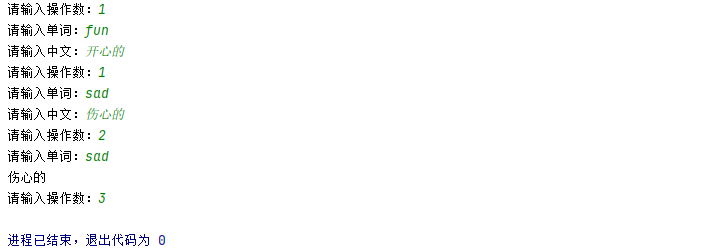2021-05-13 15:57

# 哪位大佬能帮初学者看看嘛，是用python做

• 点赞
• 写回答
• 关注问题
• 收藏
• 邀请回答

#### 2条回答默认 最新

•代码的灵魂是bug！ 2021-05-13 17:15
已采纳
``````# 记录单词
dics = {}

word = input('输入单词和中文翻译，空格分隔：')
word = word.split(' ')
dics[word] = word

def search_dic():
word = input('输入单词查找中文翻译：')
if word in dics:
return dics[word]
else:
return '未找到单词【'+word+'】的中文翻译'

if __name__ == '__main__':
while True:
num = int(input('\n请选择操作：\n1.添加单词\n2.查找单词\n3.退出程序\n'))
if num == 1:
elif num == 2:
s = search_dic()
print(s)
elif num == 3:
print('程序退出.')
break

``````
点赞 1 评论
•Norcor 2021-05-13 16:46
``````import sys

dic = {}

dic[word]=ch

def search_dic(dic,word):
return dic[word]

if __name__ == "__main__":
num = -1
while num != 3:
num = input("请输入操作数：");
if num == '1':
word = input("请输入单词：");
ch = input("请输入中文：");

elif num == '2':
word = input("请输入单词：");
print(search_dic(dic, word))
elif num == '3':
sys.exit()
else:
print("输入错误")
``````

结果如图点赞 1 评论